# The Wave – Decoding the facade

``` by Scott Brownrigg Architects
Multi Story Car Park in Cardiff Bay```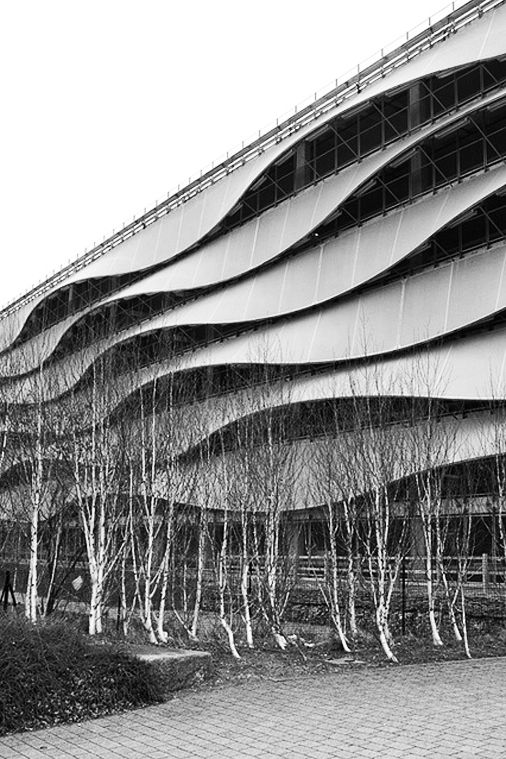Source –

https://in.pinterest.com/pin/547680004665449625/

Retrieved on 6th November,2017

The Assignment was to replicate the geometry of an interesting facade using grasshopper as the only tool. The Wave by Scott Brownrigg Architects was chosen for this exercise because of the dramatic play of fabric. Below are a few comparisons between google images and the baked rhino model.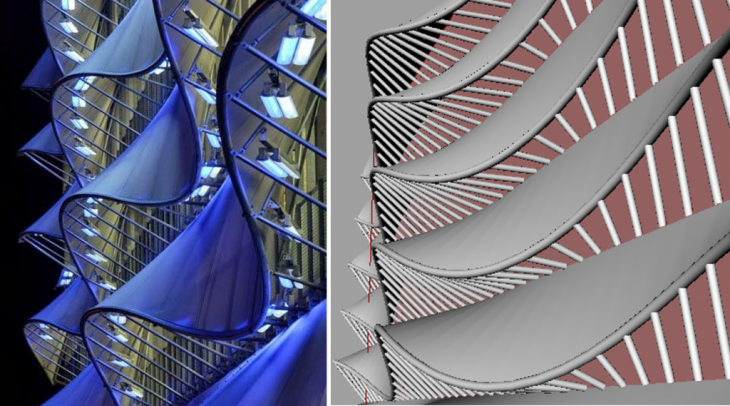A comparison of google image and the grasshopper study model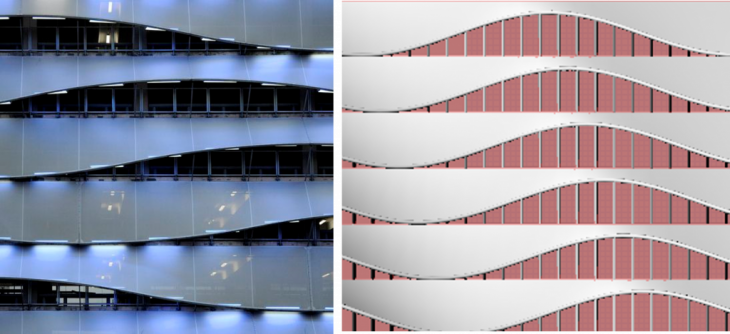A comparison of google image and the grasshopper study model

## Below are the step-by-step explanation of the process.

### 1. Base Facade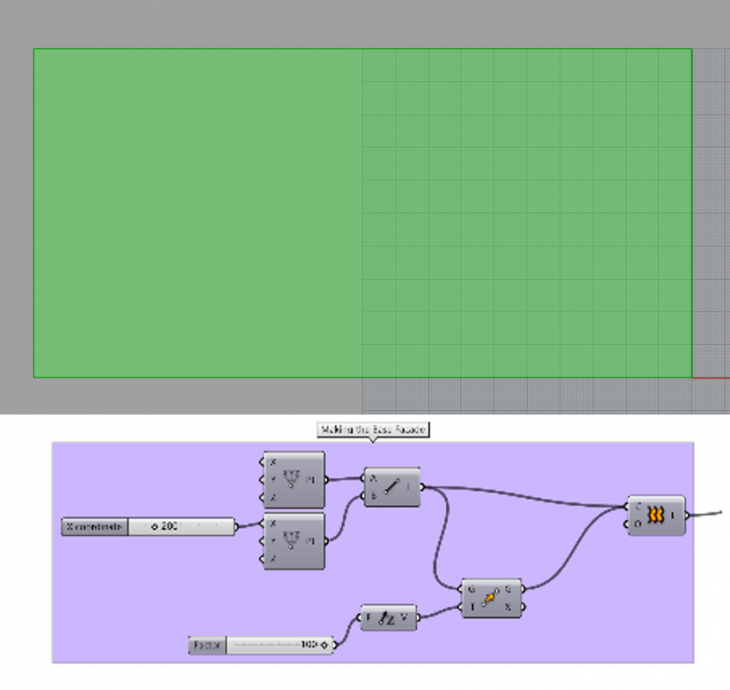• Construct 2 points
• Connect them using a line
• Move the line in Z axis
• Loft the 2 lines

### 2. Divide the Surface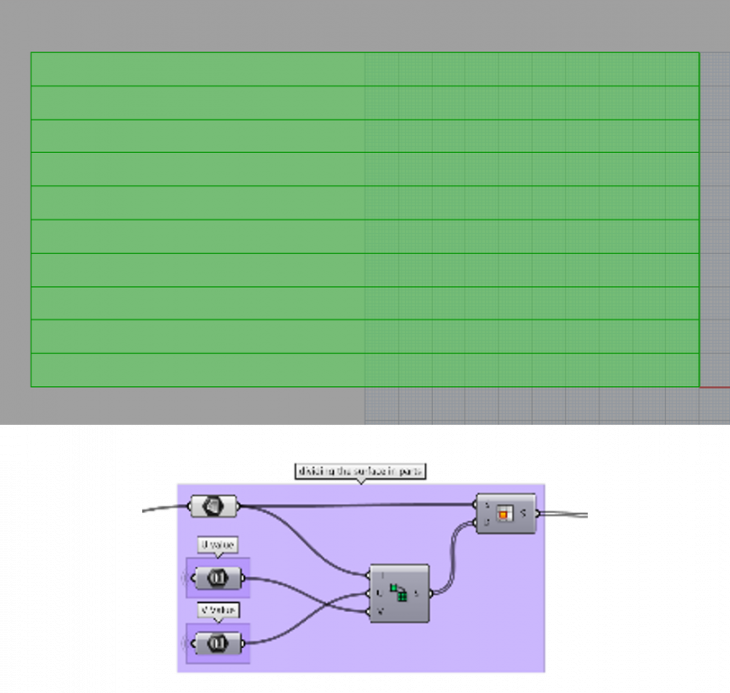• Dividing the surface using divide domain square and subsurf, into 10 equal surfaces .
• U value – 1
• V value – 10

### 3. Exploding the surfaces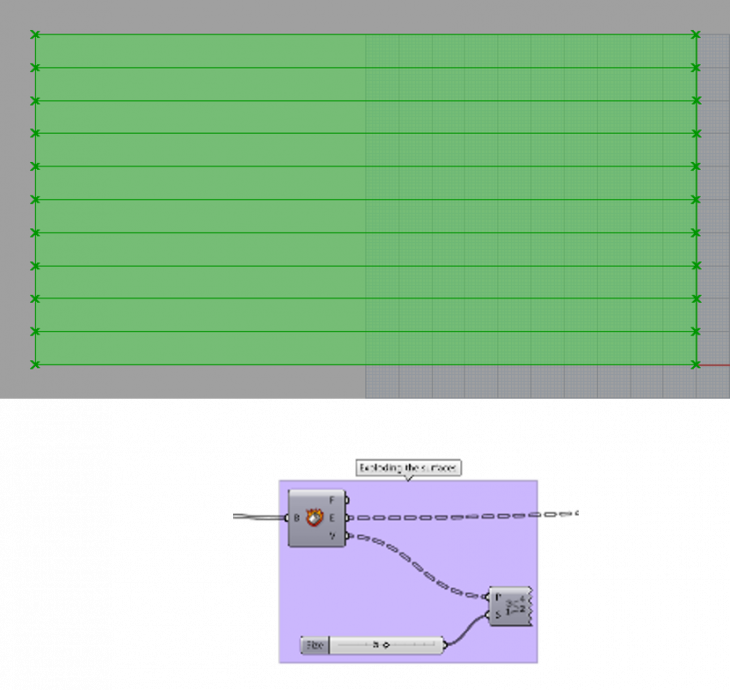• Exploding the edges using the explode component
• Making visible the vertices using the point list component .

### 4.Extracting the edges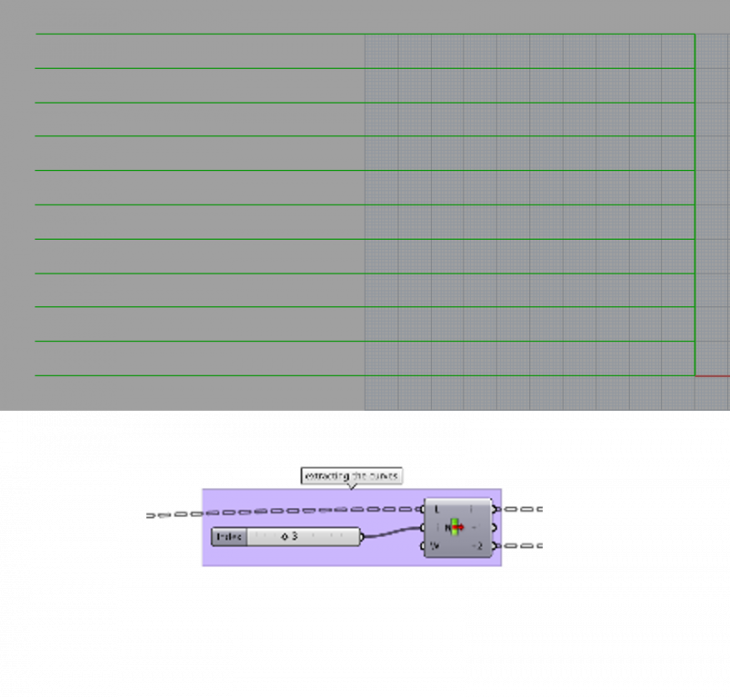• Extracting the bottom edge using the list item component after understanding the required index of the needed edge
• Also, extracting the top edge by adding 2 more parameters in the list item component which will be needed later on.

### 5. Dividing the curves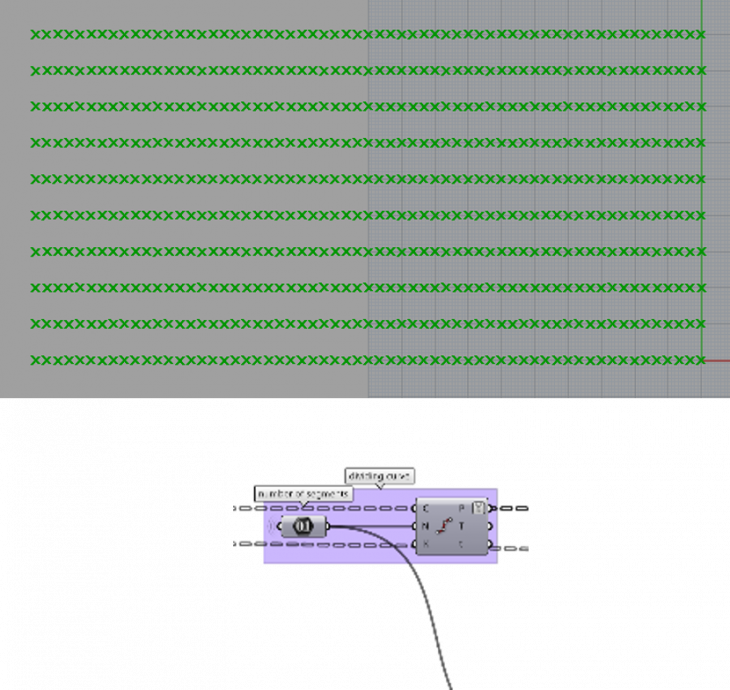• Dividing the curve into ‘x’ number of segments using the dividecurve component to use the points output.
• Simplify the points output

### 6. Sinusoidal movement in y axis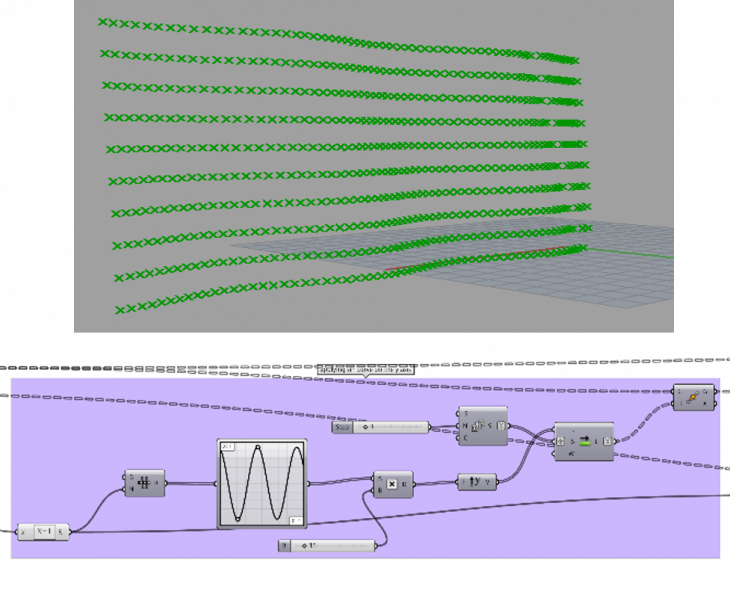• Using graph mapper (sine graph), and multiplying the result by 11 to get the amplitude of the points in the y axis.
• Using shift list component and a series of 10 numbers ( same number as the V value in the divide domain square in step 2), moving the sine curve progressively in the V direction

### 7. Sinusoidal Movement in the Z axis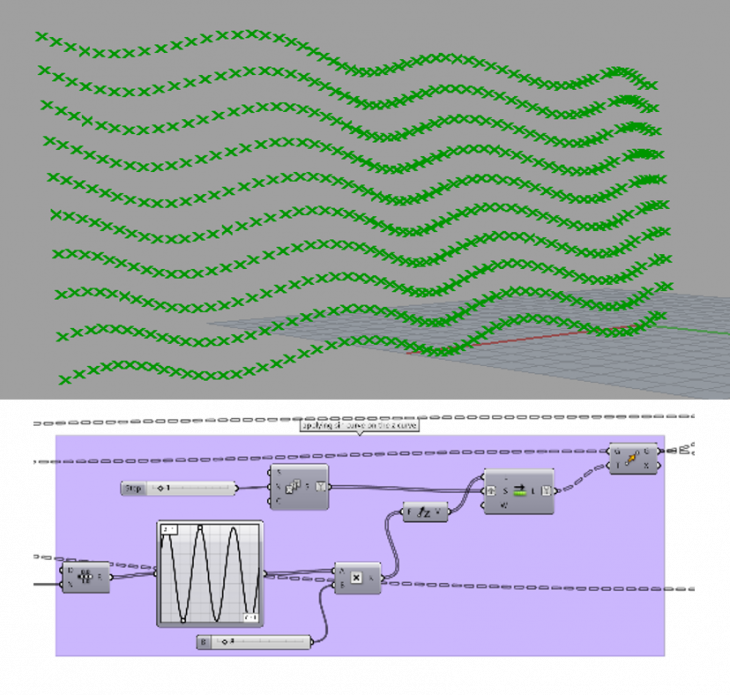• Following the same steps as in step 6, move the points in the Z axis

### 8. Interpolate curve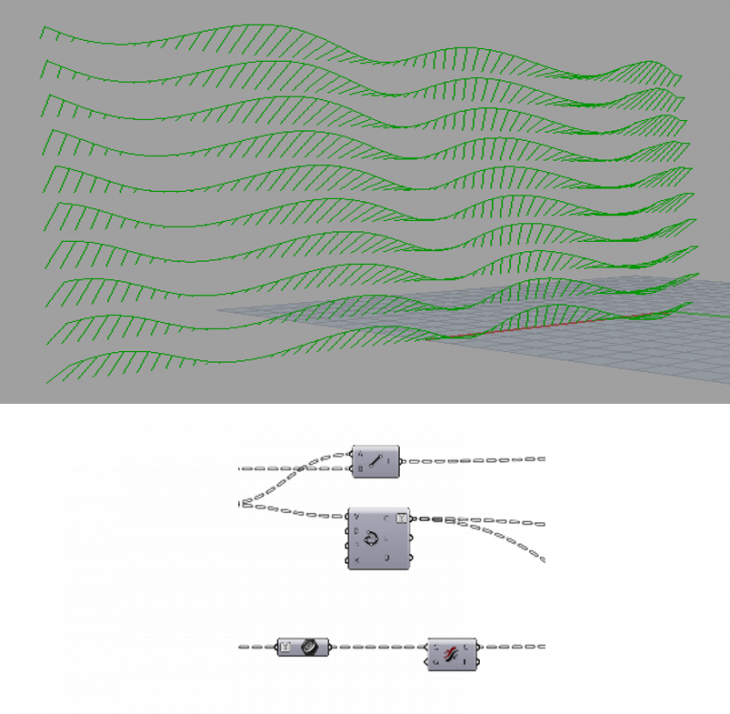• Interpolating the points moved in step 6 and 7.
• Connecting the points from step 5 and step 7

### 9. Lofting the Fabric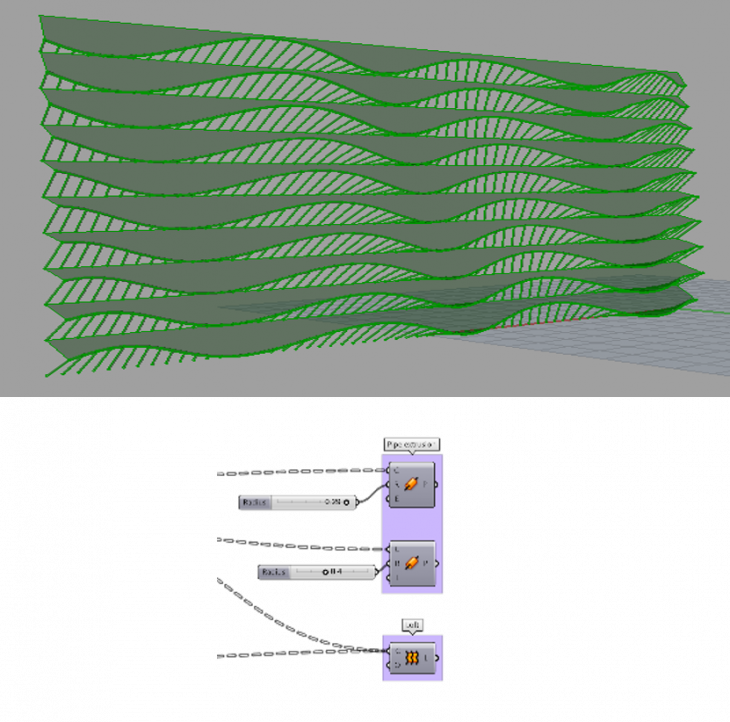• Extruding pipe along the lines generated in the last step
• Using the loft component on the top edge extracted in step 4 and the interpolated curve from step 8.

## Below is the entire grasshopper script

######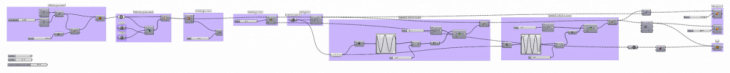‘Decoding a facade’  is a project of IaaC, Institute for Advanced Architecture of Catalonia developed at Master in Advanced Architecture, Computation Design in 2017 by:
Student: Anuj Mittal
Faculty: Rodrigo Aguirre |    Aldo Sollazzo